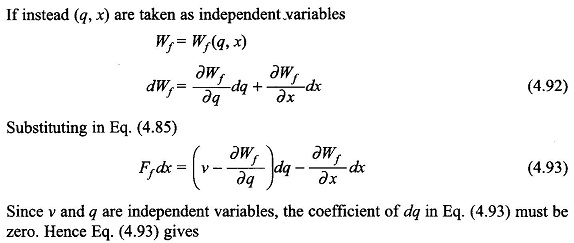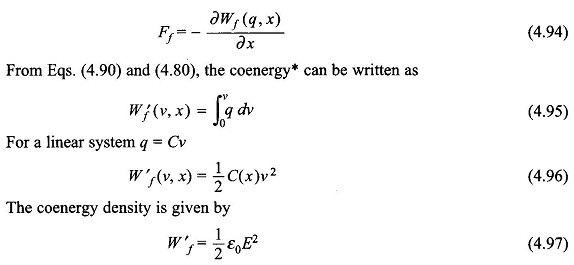# Electric Field Energy

## Electric Field Energy:

Electric Field EnergyElectromechanical energy conversion via the electric field is analogous to the magnetic field case studied earlier. Charge in the electric field is analogous to flux linkages and voltage to current in the magnetic field case.

Figure 4.18 shows a parallel plate condenser with a fixed and a movable plate. The condenser is fed from a current source. The leakage current of the condenser isrepresented outside by conductance so that the condenser’s electric field is conservative. Let us assume that the movable plate of the condenser is held fixed in position x. The electric energy input to the ideal condenser gets stored in the electric field so thatThe capacitance C is a function of configuration (position x of the movable plate) and can be expressed as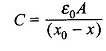where A= plate area and co = permittivity of free space. Thus Wf, the field energy is a function of two independent variables q and x, i.e.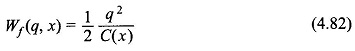The expression of Eq. (4.82) for the field energy immediately reveals that the electric field energy can be changed electrically by changing q or mechanically by changing x, i.e. moving the movable plate.

The field energy can also be written as

The energy density in the electric field can be expressed as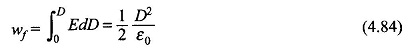where            E = electric field intensity or potential gradient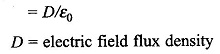## Energy Conversion

Let the movable plate of the device be now permitted to move under the action of the electric field force F1 As per the principle of energy conservation:

Mechanical energy output (work done by the field force)

= electric energy input — increase in the field energy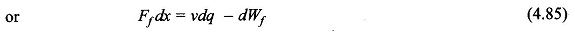Let us choose (v, x) as independent variables. ThenSubstituting Eqs (4.86) and (4.87) in Eq. (4.85) and reorganizing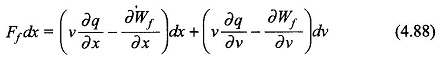Since v and x are independent variables, the coefficient of dv in Eq. (4.88) must be zero. Hence